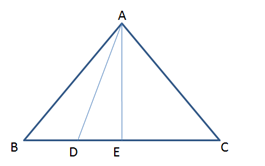Get a free home demo of LearnNext

Available for CBSE, ICSE and State Board syllabus.
Call our LearnNext Expert on 1800 419 1234 (tollfree)
OR submit details below for a call back

clear

Asked by Pranav Satti

Sep 1, 2014

Prove that 9AD2 = 7AB2

In an equilateral Δ ABC, the side BC is trisected at D. Prove that 9AD2 = 7AB2

NextGurukul Guest User

Member since Apr 1, 2017

In this solution triangle ABC and ACE is taken in place of ABC, ABE should be taken.

Raghunath Reddy

Member since Apr 11, 2014

Sol:Given:   In an equilateral triangle ΔABC. The side BC is trisected at D such that BD = (1/3) BC. To prove:  9AD2  = 7AB2 Construction:  Draw AE ⊥ BC. Proof : In a ΔABC and ΔACE AB = AC ( Given) AE = AE ( common) ∠AEB = ∠AEC = 90° ∴ ΔABC ≅ ΔACE ( For RHS criterion) BE = EC (By C.P.C.T) BE = EC = BC / 2 In a right angled triangle ADE AD2 = AE2 + DE2 ---------(1) In a right angled triangle ABE AB2 = AE2 + BE2 ---------(2) From equ (1) and (2) we obtain ⇒ AD2  - AB2 =  DE2 - BE2 . ⇒ AD2  - AB2 = (BE – BD)2 - BE2 . ⇒ AD2  - AB2 = (BC / 2 – BC/3)2 – (BC/2)2 ⇒ AD2  - AB2 = ((3BC – 2BC)/6)2 – (BC/2)2 ⇒ AD2  - AB2 = BC2 / 36 – BC2 / 4 ( In a equilateral triangle ΔABC, AB = BC = CA) ⇒ AD2 = AB2 + AB2 / 36 – AB2 / 4 ⇒ AD2 = (36AB2 + AB2– 9AB2) / 36 ⇒ AD2 = (28AB2) / 36 ⇒ AD2 = (7AB2) / 9 9AD2 = 7AB2 .

Syeda

Member since Jan 25, 2017

Answer.Given:   In an equilateral triangle ΔABC. The side BC is trisected at D such that BD = (1/3) BC. To prove:  9AD2  = 7AB2 Construction:  Draw AE ⊥ BC. Proof : In a ΔABC and ΔACE AB = AC ( Given) AE = AE ( common) ∠AEB = ∠AEC = 90° ∴ ΔABC ≅ ΔACE ( For RHS criterion) BE = EC (By C.P.C.T) BE = EC = BC / 2 In a right angled triangle ADE AD2 = AE2 + DE2 ---------(1) In a right angled triangle ABE AB2 = AE2 + BE2 ---------(2) From equ (1) and (2) we obtain ⇒ AD2  - AB2 =  DE2 - BE2 . ⇒ AD2  - AB2 = (BE – BD)2 - BE2 . ⇒ AD2  - AB2 = (BC / 2 – BC/3)2 – (BC/2)2 ⇒ AD2  - AB2 = ((3BC – 2BC)/6)2 – (BC/2)2 ⇒ AD2  - AB2 = BC2 / 36 – BC2 / 4 ( In a equilateral triangle ΔABC, AB = BC = CA) ⇒ AD2 = AB2 + AB2 / 36 – AB2 / 4 ⇒ AD2 = (36AB2 + AB2– 9AB2) / 36 ⇒ AD2 = (28AB2) / 36 ⇒ AD2 = (7AB2) / 9 9AD2 = 7AB2 .SME Approved
Like NextGurukul? Also explore our advanced self-learning solution LearnNext
Offered for classes 6-12, LearnNext is a popular self-learning solution for students who strive for excellence
Explore
Animated Video
lessons
All India
Test Series
Interactive Video
Experiments
Best-in class
books